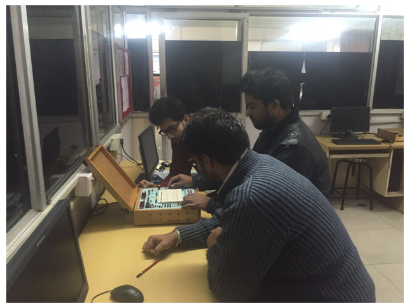Lab Room No: A308
Lab In charge: Dr. K.Sudha
Lab Attendant: Mr. Ankit Kumar
Lab Subjects: Switching Theory and Digital Logic Design-ETEE 355(V SEM)The objective of the lab is to facilitate the student with the knowledge of Logic Systems and Circuits, thereby enabling the student to obtain the platform for studying Digital Systems and Computer Architecture. It enables the students to analyze the results of logic and timing simulations and to use these simulation results to debug digital systems. This lab helps to learn to design combinational and sequential digital systems starting from a word description that performs a set of specified tasks and functions. This lab is focus on design of different type of circuits that increase understanding of course. The students design and implement a final digital project of their choice.

List of Experiment:

1. (a)To study basic gates and verify their truth tables.
(b) To study universal gates and special function gates and verify their truth tables
2. Realize all basic gates using universal gates.
3. To verify following laws of Boolean algebra :
(i) AB+ ĀB= B
(ii) Demorgon’s theorem
4. Design half adder and full adder using NAND gates/basic gates/Ex-or gates.
5. Design half subtractor and full subtractor using NAND gates/basic gates/Ex-or gates.
6. Design 2-bit magnitude comparator.
7. Analyze a 4:1 MUX using NAND gates only.
8. Design 3 bit Binary to gray code convertor.
9. Design S-R flip flop using NANDs logic gates.
10. Design 3-bit synchronous counter.